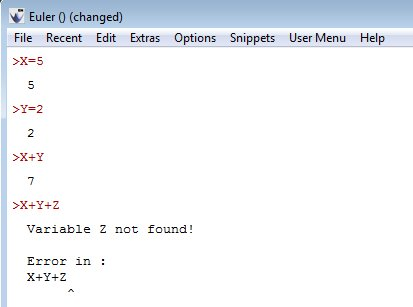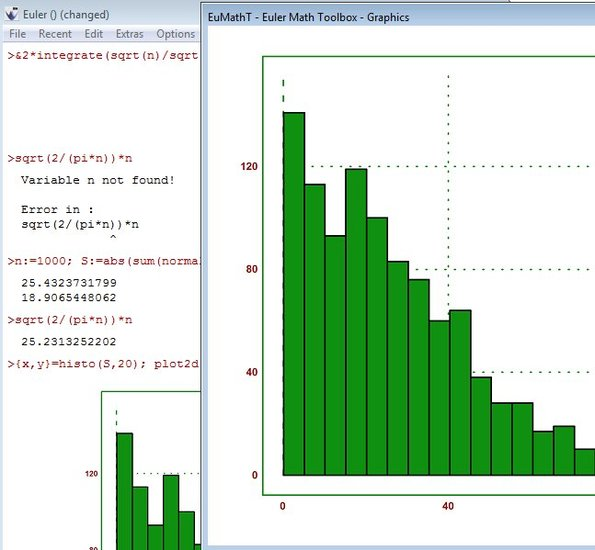Editor Ratings:
User Ratings:
[Total: 0 Average: 0]

Euler Math Toolbox is a free math calculator for Windows which you can use to perform a large number of mathematical calculations ranging from simple math problems like addition, subtraction, to more advanced calculations involving complex equations, functions, matrices and so on. When running Euler you get two windows, one where you write math problems and another one where graphs for functions will be showed, which makes this also a free graphing calculator.

Screenshot down below shows us the math problem editor of this free math calculator. Initially it’s of course totally empty. Functions that you see were added by us. Next to seeing function graphs in the additional graphing window, they also show in editor, but graphing window offers more details.We started with simple calculations, hit a few bumps, but eventually figured out how Euler Math Toolbox works. Extensive documentation is available to help you get familiar with the syntax that this free math calculator uses. A lot of example math problems can also be found in the documentation.

## Key features of Euler Math Toolbox are:

• Free and simple to setup: everything is automatically configured
• Supports both numerical and symbolic mathematical calculations
• Can be used to perform calculations of various complexity
• Works with real and/or complex numbers, intervals, vectors, matrices
• Solve algebra and calculus problems with Maxima computer algebra system
• Graphing window where you’ll be able to plot functions
• Supports exports of created graphs as PNG, Bitmap, SVG, ZIP, etc.
• Documentation: comes with a lot of tutorials, examples, and guides

Similar software: Sci9 Calculator, Free Calculator, Tibi’s Mathematical Suite, SpeQ Mathematics .

## How to perform mathematical calculations with Euler Math Toolbox: free math calculator for Windows

If you ever used Python before (Python the programming language) you will see that there’s a lot of similarities between it and the way that Euler works. For example, in order to assign values to variables, like X, you just need to type in X=5 at the prompt of the math problem editor. 5 here can be anything of course.On the image above we’ve gone a few steps further. We’ve also added a Y variable, assigned value to it and then added them both up. If you use a variable which has no value assigned to it, you’ll get an error.More complex math problems require you to know first math, and then the syntax used by this free math calculator. Everything that you need to know in order to get familiar with Euler’s syntax can be accessed by clicking on Help from the top left corner menu of the problem creator. Notice also the graphing window. To save created graphs, click File and then select the format to which you’d like to export it.

## Conclusion

Euler Math Toolbox is good for both beginners and more advanced users, but advanced mathematicians will certainly feel more at home when using it. All the basic math operations are supported and can be used, but where Euler stands out the most is when you type in very long math puzzles, which requires a lot of know-how to setup. In either case, if you’re just starting with math, or more advanced, give Euler a try and see how it goes.

1.